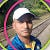# Java8: StreamsPhoto by Good Free Photos on Unsplash
`List<String> names = Arrays.asList("nuwan","","chamara","sena");List<String> validNames = names.stream().filter(s ->!s.isEmpty()).collect(Collectors.toList());`
`System.out.println( validNames.stream().collect(Collectors.joining(", ")));`
`validNames.forEach(System.out::println);`
`List<Integer> numbers = Arrays.asList(3, 2, 2, 3, 7, 3, 5);List<Integer> squaresList =numbers.stream()        .map(i -> i*i).distinct().collect(Collectors.toList());`
`squaresList.forEach(System.out::println);`
`squaresList.stream().limit(3).forEach(System.out::println);`
`squaresList.stream().sorted().forEach(System.out::println);`
`IntSummaryStatistics statistics = squaresList.stream().mapToInt(i -> i).summaryStatistics();System.out.println("Stats: "+statistics.getMax()+" "+ statistics.getSum()+" "+ statistics.getAverage());`
`System.out.println("Count: "+squaresList.parallelStream().count());`

--

--## Nuwan Zen

Sometimes A software Engineer, sometimes a support engineer, sometimes a devops engineer, sometimes a cloud engineer :D That’s how the this life goes!